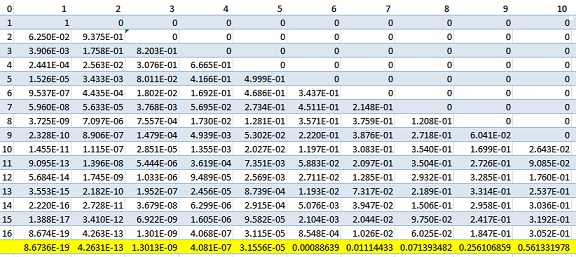# Practical Inevitability of Empty Spaces

### Problem### N. N. Taleb's General Solution

We start with the general. The $\textit{weak compositions}$ of a positive integer $n$ into $d$ ordered parts treating $0$ as a significant addend (e.g. $0+3$ is different from $3+0)$ is given by the vector $C_{p,k}.$ The number of such weak compositions will be:

$\displaystyle \frac{(d+n-1)!}{(d-1)! n!}$

Let $\mathcal{M}_{(n,\overset{\rightharpoonup }{p})}(\overset{\rightharpoonup }{\chi _i})$ be the probability mass function for the multinomial distribution for $n$ independent trials and probability vector $\overset{\rightharpoonup }{p}$, of dimension $d$, with vector of realizations $\overset{\rightharpoonup }{\chi _i}$ comprising the $C_{p,k}$ compositions. In the specific problem with 16 squares and 16 throws, $(\overset{\rightharpoonup }{\chi _i})$ would be $\overset{\rightharpoonup }{\chi_1}=\underbrace{(16,0,\ldots,0)}_{d=16}$, $\overset{\rightharpoonup }{\chi_2}=\underbrace{(15,1,\ldots,0)}_{d=16}$, ..., $\overset{\rightharpoonup }{\chi}_{300,540,195}= \underbrace{(0,0,\ldots,16)}_{d=16}$ with the probability vector $\overset{\rightharpoonup }{p}= \left(p_1,\ldots,p_{16}\right)=\left(\frac{1}{16},\ldots,\frac{1}{16}\right)$, etc.

More specifically,

$\displaystyle \mathcal{M}_{(n,\overset{\rightharpoonup }{p})}(\overset{\rightharpoonup }{\chi _i})= n! \prod _{j=1}^d \frac{p_j^{(\overset{\rightharpoonup }{\chi _i})_j}}{(\overset{\rightharpoonup }{\chi _i})_j!}$

We finally have, using $z(.)$ the $\#$ of zeros in the vector $\chi _i$.

$\displaystyle \mathbb{P}(M\geq m)=\sum _{i=1}^{\frac{(d+n-1)!}{(d-1)! n!}} \mathbb{1}_{z( \overset{\rightharpoonup }{\chi _i})\geq m}\mathcal{M}_{\left(n,\overset{\rightharpoonup }{p}\right)}\left(\overset{\rightharpoonup }{\chi _i}\right)$

On our example, it can be compressed to a single line using Mathematica code with the package Combinatorica:

\displaystyle \begin{align} &\chi =\text{Compositions}(16,16);\\ &\sum _{i=1}^{\text{Length}[\chi ]} \text{PDF}[\text{MultinomialDistribution}[16,p],\chi [[i]]]\times\\ &\qquad\qquad\text{Boole}[\text{Length}[\text{Select}[\chi [[i]],\text{\#1}=0\&]]>8] \end{align}

### Alternative, computationally efficient Solution

The solution in Eq.1, while mathematically elegant, is not the most computationally efficient, as the number of components of $C_{p,k}$ exceed 300 million.

We first observe that the vectors $(15,1,0,\ldots,0)$ and $(15, 0,1,\ldots,0)$ have the same probability of occurring. We can thus simply focus on partitions of $n$ ignoring $d$. So we have the matrix $\kappa= \bigg((16), (15,1),(14,1,1),\ldots ,(1,1,\ldots,1)\bigg)$. $\kappa$ has 231 vector columns $\vec{\kappa_1}, \vec{\kappa_2},...$, etc.

Next we compute the number of permutations of each the corresponding $\vec{\kappa_i}$ if we add the zeros, say $(16,0,\ldots,0)$,$(0,16,\ldots,0)$, etc. $\vec{\lambda}=(16, 240, 240, 1680, 240, 3360,...)$

Next we select only those $\vec{\kappa_i}$ that have less than $8$ with a vector of indicator functions. $\vec{I}=(1,1,...0)$

Finally we build a table of probability masses for each $\kappa_i$ with $\kappa_i^{'}$ if we completed the corresponding partition with zeros to make it of dimension 16. For instance, $\kappa_1^{'}=\underbrace{(16,0,\ldots,0)}_{16}$, $\kappa_2^{'}=\underbrace{(15,1,\ldots,0)}_{16}$, etc.

$\displaystyle \vec{M}=\bigg(\mathcal{M}_{16,\vec{p}}(\vec{\kappa_1^{'}}), \mathcal{M}_{16,\vec{p}}(\vec{\kappa_2^{'}}),\ldots,\bigg)$.

We note that all vectors $\vec{I}$, $\vec{M}$, and $\vec{\lambda}$ are of dimension 231, which makes the next operation extremely quick. The probability of having 8 or more blank squares in 16 throws is therefore:

$\displaystyle \sum_{i=1}^{231} \vec{I}_i \times \vec{M}_i \times \vec{\lambda}_i\approx 0.0713935$

### Chris Ball's Recurrence

Let $P(I,N)$ denote the probability $N$ squares being hit after $I$ darts have been thrown. Then

\begin{align}P(i,n)&= P(i-1,n-1)\times P(\text{hitting one of the vacant squares})\\ &\qquad+ P(i-1,n)\times P(\text{hitting one of the already hit squares})\\ &=P(i-1,n-1)\cdot\frac{17-n}{16}+P(i-1,n)\cdot\frac{n}{16}. \end{align}

Speculatively, $P(0,0)=1$ as the probability of hitting nothing when throwing nothing; $P(i,0)=0,$ $i\gt 0,$ as the stipulation that every single dart hits the board; $P(0,n)=0,$ $n\gt 0,$ as the probability of hitting anything when throwing nothing.

The sought probability is $\displaystyle \sum_{n=1}^8P(16,n).$

In the spreadsheet below (mine first but following Chris' example), the number of thrown darts are on the vertical axis, the number of the hit squares are on the horizontal axis. The last row is the cumulative probability of $16$ darts hitting at most the specified number of squares. So that, for example, the probability that $8$ squares or less were hit is about $0.07139,$ more than $7\%.$The probability of $7$ empty squares is $0.2561$ and that of having $6$ empty squares is $0.5613.$ In other words, $37.5\%$ of the the squares will be missed by the darts with probability of more than $56\%.$

### Long Huynh Huu's Recurrence

Let $d = 16$ be the number of squares and $n = 16$ the number of darts.

1. We may perform a case-distinction by the number $k$ of squares being hit a at least one dart. $k = 1,...,\lfloor d/2 \rfloor$.
2. Given $k$, there are $\binom{d}{k}$ choices of $k$ squares to hit
3. We count $k!S(n,k)$ ways to map each dart to one of the $k$ chosen squares -- surjectively
4. In total there are $d^n$ ways to distribute the darts into the squares.

$\displaystyle \frac{\sum_{k=1}^{\lfloor \frac{d}{2} \rfloor} \frac{d!}{(d-k)!}S(n,k)}{d^n}.$

The Stirling number $S(n,k)$ counts how many ways there are to partition an $n$-set into $k$-blocks.

The blocks are not ordered, however the elements of the $n$-set are distinguished. We have

\begin{align} &\cdot\; S(n,k) = kS(n-1,k) + S(n-1,k-1),\\ &\cdot\; S(n,0) = 0,\\ &\cdot\; S(n,1) = 1,\\ &\cdot\; S(n,k) = 0, \text{ if } k \gt n. \end{align}

Implementation in Julia:

function S(n,k)
if k > n || k == 0
0
elseif k == 1
1
else
k*S(n-1,k) + S(n-1,k-1)
end
end

function CTK_probability(d,n,m=div(d,2))
sum(map(k -> prod(Array{BigInt}(d-k+1:d))*S(BigInt(n),BigInt(k)), 1:m))
// (BigInt(d)^n)
end


Result: 7.139%

### Acknowledgment

This is a follow-up to the previous discussion on the occurrence of clusters in similar experiments.

Steve Phelps has created an eluminating GeoGebra simulation.